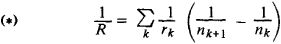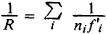# Curvature of Image Field

The following article is from The Great Soviet Encyclopedia (1979). It might be outdated or ideologically biased.

## Curvature of Image Field

one of the aberrations of optical systems; it consists in a sharp image of a plane object being formed on a curved surface rather than on a plane, as it should be in an ideal system. If the lenses forming part of a centered system have spherical refracting surfaces of radii rk (k is the number of the surface in the path of the light ray) and if astigmatism has been corrected in the system, then the image of a plane perpendicular to the axis of the system is a sphere. Its radius R is given by the equationwhere nk and nk + 1 are the refractive indexes of the media in front and behind the kth refracting surface. For thin-lens systems, equation (*) reduces towhere fi is the focal length of the ith lens and ni is the refractive index of the material of the lens. The curvature of image field is corrected in complex optical systems (for example, in photographic objectives) by combining lenses with surfaces of various curvatures such that the right-hand side of equation (*) equals zero (the Petzval condition).

### REFERENCES

Tudorovskii, A. I. Teoriia opticheskikh priborov, 2nd ed. Moscow-Leningrad, 1948.
Sliusarev, G. G. Metody rascheta opticheskikh sistem, 2nd ed. Leningrad, 1969.
The Great Soviet Encyclopedia, 3rd Edition (1970-1979). © 2010 The Gale Group, Inc. All rights reserved.
Site: Follow: Share:
Open / Close